Next: Microscopic and macroscopic systems Up: Introduction Previous: Thermodynamics

## The need for a statistical approach

It is necessary to emphasize from the very outset that this is a difficult subject. In fact, this subject is so difficult that we are forced to adopt a radically different approach to that employed in other areas of Physics.

In all of the Physics courses which you have taken up to now, you were eventually able to formulate some exact, or nearly exact, set of equations which governed the system under investigation. For instance, Newton's equations of motion, or Maxwell's equations for electromagnetic fields. You were then able to analyze the system by solving these equations, either exactly or approximately.

In thermodynamics we have no problem formulating the governing equations. The motions of atoms and molecules are described exactly by the laws of quantum mechanics. In many cases, they are also described to a reasonable approximation by the much simpler laws of classical mechanics. We shall not be dealing with systems sufficiently energetic for atomic nuclei to be disrupted, so we can forget about nuclear forces. Also, in general, the gravitational forces between atoms and molecules are completely negligible. This means that the forces between atoms and molecules are predominantly electromagnetic in origin, and are, therefore, very well understood. So, in principle, we could write down the exact laws of motion for a thermodynamical system, including all of the inter-atomic forces. The problem is the sheer complexity of this type of system. In one mole of a substance (e.g., in twelve grams of carbon, or eighteen grams of water) there are Avagadro's number of atoms or molecules. That is, about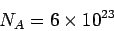particles, which is a gigantic number of particles! To solve the system exactly we would have to write down about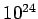coupled equations of motion, with the same number of initial conditions, and then try to integrate the system. Quite plainly, this is impossible. It would also be complete overkill. We are not at all interested in knowing the position and velocity of every particle in the system as a function of time. Instead, we want to know things like the volume of the system, the temperature, the pressure, the heat capacity, the coefficient of expansion, etc. We would certainly be hard put to specify more than about fifty, say, properties of a thermodynamic system in which we are really interested. So, the number of pieces of information we require is absolutely minuscule compared to the number of degrees of freedom of the system. That is, the number of pieces of information needed to completely specify the internal motion. Moreover, the quantities which we are interested in do not depend on the motions of individual particles, or some some small subset of particles, but, instead, depend on the average motions of all the particles in the system. In other words, these quantities depend on the statistical properties of the atomic or molecular motion.

The method adopted in this subject area is essentially dictated by the enormous complexity of thermodynamic systems. We start with some statistical information about the motions of the constituent atoms or molecules, such as their average kinetic energy, but we possess virtually no information about the motions of individual particles. We then try to deduce some other properties of the system from a statistical treatment of the governing equations. If fact, our approach has to be statistical in nature, because we lack most of the information required to specify the internal state of the system. The best we can do is to provide a few overall constraints, such as the average volume and the average energy.

Thermodynamic systems are ideally suited to a statistical approach because of the enormous numbers of particles they contain. As you probably know already, statistical arguments actually get more exact as the numbers involved get larger. For instance, whenever I see an opinion poll published in a newspaper, I immediately look at the small print at the bottom where it says how many people were interviewed. I know that even if the polling was done without bias, which is extremely unlikely, the laws of statistics say that there is a intrinsic error of order one over the square root of the number of people questioned. It follows that if a thousand people were interviewed, which is a typical number, then the error is at least three percent. Hence, if the headline says that so and so is ahead by one percentage point, and only a thousand people were polled, then I know the result is statistically meaningless. We can easily appreciate that if we do statistics on a thermodynamic system containingparticles then we are going to obtain results which are valid to incredible accuracy. In fact, in most situations we can forget that the results are statistical at all, and treat them as exact laws of Physics. For instance, the familiar equation of state of an ideal gas,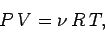is actually a statistical result. In other words, it relates the average pressure and the average volume to the average temperature. However, for one mole of gas the statistical deviations from average values are only about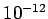, according to the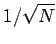law. Actually, it is virtually impossible to measure the pressure, volume, or temperature of a gas to such accuracy, so most people just forget about the fact that the above expression is a statistical result, and treat it as a law of Physics interrelating the actual pressure, volume, and temperature of an ideal gas.Next: Microscopic and macroscopic systems Up: Introduction Previous: Thermodynamics
Richard Fitzpatrick 2006-02-02